Points to Remember: Understanding Quadrilaterals

# Points to Remember: Understanding Quadrilaterals Notes | Study Mathematics (Maths) Class 8 - Class 8

## Document Description: Points to Remember: Understanding Quadrilaterals for Class 8 2022 is part of Mathematics (Maths) Class 8 preparation. The notes and questions for Points to Remember: Understanding Quadrilaterals have been prepared according to the Class 8 exam syllabus. Information about Points to Remember: Understanding Quadrilaterals covers topics like and Points to Remember: Understanding Quadrilaterals Example, for Class 8 2022 Exam. Find important definitions, questions, notes, meanings, examples, exercises and tests below for Points to Remember: Understanding Quadrilaterals.

Introduction of Points to Remember: Understanding Quadrilaterals in English is available as part of our Mathematics (Maths) Class 8 for Class 8 & Points to Remember: Understanding Quadrilaterals in Hindi for Mathematics (Maths) Class 8 course. Download more important topics related with notes, lectures and mock test series for Class 8 Exam by signing up for free. Class 8: Points to Remember: Understanding Quadrilaterals Notes | Study Mathematics (Maths) Class 8 - Class 8
 1 Crore+ students have signed up on EduRev. Have you?

1. A simple closed curve made of only line segments is called a polygon.
2. If all the sides of a polygon are equal and all angles are also equal, it is said to be a regular polygon.
3. An equilateral triangle is a regular polygon of 3 sides and square is a regular polygon of 4 sides.
4. The sum of the exterior angles of a polygon is always 360°.
5. A quadrilateral in which a pair of opposite sides are parallel is called a trapezium.
6. If both the pairs of opposite sides of a quadrilateral are parallel then it is called a parallelogram.
7. A parallelogram that has all four sides equal, is called a rhombus.
8. A parallelogram that has all its angles at right angles, is called a rectangle.
9. A parallelogram with all sides and all angles equal, is a square.
10. A kite is a special quadrilateral in which one pair of adjacent sides are equal to each other and other pair of adjacent sides are equal to each other.

Remember
1. A triangle does not have a diagonal as each vertex is adjacent to the other two.
2. If all sides of a quadrilateral are equal, its diagonals are perpendicular.
3. If all angles of a quadrilateral are equal, diagonals are equal.
4. If opposite sides of a quadrilateral are parallel and equal, diagonals bisect each other.
5. In a kite only the obtuse angles opposite to each other are equal not the acute angles.
6. In a trapezium the angels are not equal unless it is an isosceles trapezium.
7. A square is also a trapezium, rectangle and rhombus.

WE KNOW THAT
A curve which does not cut itself is called an open curve whereas a curve which cuts itself is called a closed curve. The closed curves which do not cross themselves are called simple curves. A curve (in mathematics) can be straight also.

POLYGONS
A simple closed curve made up of only the line segments is called a polygon. The simplest polygon is a triangle which is made up of 3 line segments. Let us classify the polygons according to the number of sides (or vertices) they have:

 Number of sides or vertices Name of the polygon 3 Triangle 4 Quadrilateral 5 Pentagon 6 Hexagon 7 Heptagon 8 Octagon 9 Nonagon 10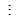Decagonn n-gon

The line connecting any two non-consecutive vertices is called a diagonal. Convex polygons have no portion of their diagonals in their exteriors. Thus a polygon each of whose interior angles is less than 180°, is called a convex polygon, otherwise it is concave polygon.

REGULAR AND IRREGULAR POLYGONS
A regular polygon has sides of equal length and angles of equal measure, i.e. a regular polygon is both ‘equiangular’ and ‘equilateral’.
Examples: (i) An equilateral triangle has sides of equal length and angles of equal measure.
Therefore, it is a regular polygon.
(ii) A square has sides of equal length and angles of equal measure. So, it is a regular polygon.
(iii) A rectangle is equiangular but not equilateral. Therefore, it is not a regular polygon.
ANGLE SUM PROPERTY
(i) The sum of the measures of angles of a quadrilateral is 360°.
(ii) If the sides of a quadrilateral are produced in order, the sum of four exterior angles so formed is 360°.
Note: If we denote some angles by ∠1, ∠2, ∠3, etc. Then their corresponding measures are m∠1, m∠2, m∠3, etc.

1. Trapezium
A quadrilateral having a pair of parallel sides is called a trapezium. In the figure, ABCD is a trapezium in which AD || BC. AB and DC are non-parallel sides. In case the non-parallel sides are equal then the trapezium is called an isosceles trapezium.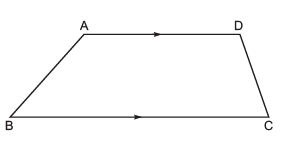In the figure, PQRS is an isosceles trapezium in which PQ || RS and non-parallel sides PS = QR.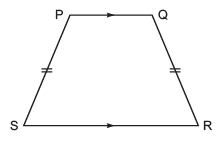A kite is a quadrilateral having two distinct consecutive pairs of sides of equal lengths. In the figure ABCD is a kite having AB = AC and BD = CD.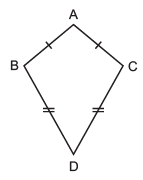2. Parallelogram
A quadrilateral having its opposite sides parallel is called a parallelogram. In the figure, ABCD is parallelogram such that AB || CD and AD || BC.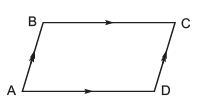PROPERTIES OF A PARALLELOGRAM
(i) The opposite sides of a parallelogram are parallel.
(ii) The opposite sides of a parallelogram are equal.
(iii) The opposite angles of a parallelogram are equal.
(iv) The adjacent angles of a parallelogram are supplementary.
(v) The diagonals of a parallelogram bisect each other; but they are not equal.
Note: I. The four sides and four angles of a parallelogram are called its elements.
II. Two pairs of opposite sides are AB || CD and BC || AD. ∠A and ∠C are a pair of opposite angles and ∠B and ∠D form another pair of opposite angles. ∠A and ∠D are adjacent angles. ∠B and ∠A; ∠B and ∠C; ∠C and ∠D, etc. are pairs of adjacent angles. Similarly,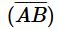and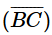are adjacent sides, other pairs of adjacent sides areand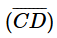;and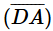;and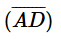.

Solved Question:
Question: A rectangle is also a kind of parallelogram discuss why?
Solution: A rectangle is also a parallelogram because all its opposite sides are parallel to each other. Despite forming an angle of 90°, rectangle due to its parallel sides can be said to be a parallelogram.

Question: A parallelogram FAST is shown in the figure. Find the missing angles.Parallelogram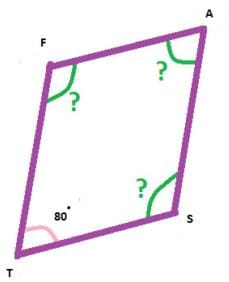Solution: FAST is a parallelogram. Let us first recollect the angle related properties of a parallelogram.
Opposite angles are equal.
Adjacent angles are supplementary.
Using the first property, let us find the value of  ∠FAS
∠FAS is opposite to ∠FTS, so ∠FAS shall be 80°

Now let us use the 2nd angle property, which says adjacent angles are supplementary.
According to this property, ∠FAS + ∠AST = 180°
80° + ∠AST = 180°
∠AST = 180-80
= 100°
For finding the value of ∠AFT we need to use the opposite angle property.
∠AFT =∠AST
∠AST = 100°
So, ∠AFT = 100°

Question: Find the value of the following angles in the rhombus ABCD:
a. ∠XAB
b. ∠XCB and
c. ∠AXB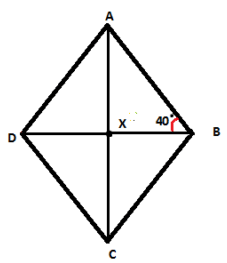Solution:
a) DXB is the diagonal that bisects the ∠ABC

Since ∠ABX = 40 °, ∠ABC = 2× 40 = 80°

Now in a rhombus, the sum of adjacent angles is 180° so ∠ABC+∠BAD = 180°

80° + ∠BAD = 180°

∠BAD is bisected by diagonal,AXC, so ∠ XAB = 50 °

b) ∠XAB is opposite to ∠ XCB, therefore ∠XCB=50°

c) ∠AXB is formed by the diagonal that perpendicularly bisect each other, therefor ∠AXB is 90°.

The document Points to Remember: Understanding Quadrilaterals Notes | Study Mathematics (Maths) Class 8 - Class 8 is a part of the Class 8 Course Mathematics (Maths) Class 8.
All you need of Class 8 at this link: Class 8

## Mathematics (Maths) Class 8

187 videos|323 docs|48 tests
 Use Code STAYHOME200 and get INR 200 additional OFF

## Mathematics (Maths) Class 8

187 videos|323 docs|48 tests

Track your progress, build streaks, highlight & save important lessons and more!

,

,

,

,

,

,

,

,

,

,

,

,

,

,

,

,

,

,

,

,

,

;